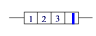#### The Resistor Colour Code

• The code is a convenient way to tell the resistance of a resistor.
•  It is also used for other components such as capacitors
•  Coloured bands are placed on a resistor.  Each band has a numerical value.
•  Bands are read from left to right.1 - First colour band = 1st digit
2 - Second colour Band = 2nd digit
3 - Third colour Band  = Multiplier
The last band (usually separated from the others) = the tolerance (how accurate the R value is)

Resistors colour codes - The following table should be memorized

 COLOUR OF BAND FIRST BAND SECOND BAND Multiplier Tolerance BLACK 0 0 1 BROWN 1 1 10 RED 2 2 100 ORANGE 3 3 1,000 YELLOW 4 4 10,000 GREEN 5 5 100,000 BLUE 6 6 1,000,000 VIOLET 7 7 10,000,000 GRAY 8 8 100,000,000 WHITE 9 9 1,000,000,000 SILVER + or - 10% GOLD + or - 5%

EXAMPLE:  What is the value of resistance of a resistor with the following coloured bands:

Yellow, green, red, gold
Solution
Yellow = 4   à  first number = 4
Green  = 5   à    second number = 5
Red (in the third band) = X100
Therefore: THE VALUE OF THE RESISTOR IS 45x100 = 4,500 ohms   OR 4.5kΩ (4.5 KILO-OHMS)

Since the last band is gold, the accuracy of this resistor is ± 5%.
This means that it can have a value that can go from a high value of  [4500 + 5%*(4500)] = [4500 + 225] = 4725 Ω to a low value of  .....     [4500 – 5%*(4500) ] = [4500  - 225] = 4275 Ω